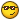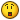# Automatic .rar and .par

## Recommended Posts

```#include <File.au3>
#include <Array.au3>

Dim \$filter
Dim \$rars
Dim \$pars
\$n=0
\$path=(@ScriptDir)

;MsgBox(0, "", \$path)

\$filter="*.rar"
\$filter="*.part001.rar"
\$filter="*.par"

\$rar=_FileListToArray(\$path, \$filter, 0)
\$end=Ubound(\$rar)

Do
\$n=\$n+1
\$teststring=_ArrayToString(\$rar, @CR, \$n, \$n)
\$found=StringInStr (\$teststring, ".part")

Select
case \$found=0
EndSelect

Until \$n=\$end
_ArraySort(\$rars)
_ArrayDelete(\$rars, 0)
_ArraySort(\$rars)
\$rar=\$rars
\$n=0

\$par=_FileListToArray(\$path, \$filter, 0)

\$end=Ubound(\$par)

Do
\$n=\$n+1
\$teststring=_ArrayToString(\$par, @CR, \$n, \$n)
\$found=StringInStr (\$teststring, ".vol")

Select
case \$found=0
EndSelect

Until \$n=\$end
_ArraySort(\$rars)
_ArrayDelete(\$pars, 0)
_ArraySort(\$pars)
\$par=\$pars
\$n=0

\$part01=_FileListToArray(\$path, \$filter, 0)

Run ("C:\Program Files\QuickPar\QuickPar.exe" & " " & \$path & "\" & \$par); this will not work

; .rar is no problem becouse rar.exe handles added filenames with : rar.exe e filename dir_to_extract_to```

What this program does, is find al .part001.rar and .rar and .par files in the current directory.

I'm making a automatic unpacking script.

This doesn't work becouse Quickpar doesnt handle added filenames.

What i'm searching for is a command, is that handles files the same way as being double clicked on in explorer.

Then i can make this workPS i'm not an elegant coder.

##### Share on other sites

Run (@COMSPEC & ' /c start file.rar' ...)

or

DllCall & ShellExecute & command Open

Try search for it in forum and HelpFile...

##### Share on other sites

You could do what I do and use either DlDone or rarslave. These can be tricky to find on the internet (especially DlDone), but you can find them in the news groups.

Give a man a script; you have helped him for today. Teach a man to script; and you will not have to hear him whine for help.AutoIt4UE - Custom AutoIt toolbar and wordfile for UltraEdit/UEStudio users.AutoIt Graphical Debugger - A graphical debugger for AutoIt.SimMetrics COM Wrapper - Calculate string similarity.

##### Share on other sites

Run (@COMSPEC & ' /c start file.rar' ...)

or

DllCall & ShellExecute & command Open

Try search for it in forum and HelpFile...

Thank you for your reply, alltough i'm provicient at english, its not my native language.

So i didnt know what to search for on the forum and the helpfile, now i do##### Share on other sites

You could do what I do and use either DlDone or rarslave. These can be tricky to find on the internet (especially DlDone), but you can find them in the news groups.

I like to do this in my own script, its a nice exerciseBut thanks for the help.

I will post the script when its doneEdited by WhiteCrow
##### Share on other sites

You probably have the spaces in path problem. Change the code as follows.

`Run('C:\Program Files\QuickPar\QuickPar.exe' & ' "' & \$path & '\' & \$par & '"'); this will work`

Works fine for me after the change.

Did you know that there is a Par2 command line program. Search for Par2Cmd on google or check it out here:

Par2Cmd in Par2 Forums

Post your code as you go along. I am interested in your future plans.

What I would like is for it to sit in the background monitoring for new files, checking them, unpacking them and deleting them when done. That is sort of what DlDone does, but it's user interface is not very clean.

Edited by Stumpii

Give a man a script; you have helped him for today. Teach a man to script; and you will not have to hear him whine for help.AutoIt4UE - Custom AutoIt toolbar and wordfile for UltraEdit/UEStudio users.AutoIt Graphical Debugger - A graphical debugger for AutoIt.SimMetrics COM Wrapper - Calculate string similarity.

##### Share on other sites

Thanks for the advice stumpii, but i figured it out , my script works realy well nowIt has an ini to store all defaults, im rather proud of itNext thing to do is to add it to the contextmenu of windows..

```#include <File.au3>
#include <Array.au3>
Opt("WinTextMatchMode", 2)
Opt("WinSearchChildren", 1)

Dim \$filter
Dim \$temprar
Dim \$temppar
Dim \$failed 
\$count=0
\$inifilenpath=@ScriptDir & "\" & "parrar.ini"

\$gogogo=1
\$gogo=0
\$n=0

endif

\$winrarpath=FileOpenDialog("What is the location of Winrar ?", "", "Rar.exe (Rar.exe)", 1 + 2, "Rar.exe" )
IniWrite ( \$inifilenpath, "WinRarDir", "WinRar", \$winrarpath)
endif

\$yesno=msgbox(4, "Delete or Not", "Do you want PaRRaR to ask if you want to delete the processdir after each run ?" & @CR & "This option also gives you some more stats when your done processing.")
if \$yesno = 6 then
Iniwrite ( \$inifilenpath, "DeleteDir", "Delete", "yes" )
else
Iniwrite ( \$inifilenpath, "DeleteDir", "Delete", "no" )
endif

MsgBox(0, "Settings", "Settings Done, written in parrar.ini (same place as parrar.exe)" & @CR & "Change the .ini if you like, or delete parrar.ini to reset the settings, and be asked these questions again.")
endif

\$path=FileSelectFolder ( "Wich directory do you want to process the Par and Rar files from ? ", \$DownDir)
\$deleteyes=\$path
\$path=StringReplace ( \$path, " ", '"' & " " & '"')

\$filter="*.rar"
\$filter="*.part001.rar"
\$filter="*.par"
\$filter="*.part01.rar"
\$filter="*.part1.rar"
\$filter="*.001"

\$rar=_FileListToArray(\$path, \$filter, 0)

if \$rar <> "" then

\$end=Ubound(\$rar)
Do
\$n=\$n+1
\$teststring=_ArrayToString(\$rar, @CR, \$n, \$n)
\$found=StringInStr (\$teststring, ".part")

Select
case \$found=0
EndSelect

Until \$n=\$end -1
\$rar=\$temprar
\$n=0

endif

\$par=_FileListToArray(\$path, \$filter, 1)

;_ArrayDisplay ( \$par, "pars" )

if \$par <> "" then

\$end=Ubound(\$par)

Do
\$n=\$n+1
\$teststring=_ArrayToString(\$par, @CR, \$n, \$n)
\$found=StringInStr (\$teststring, ".vol")

Select
case \$found=0
EndSelect

Until \$n=\$end -1
_ArraySort(\$temppar)
\$par=\$temppar
\$n=0

endif

\$part001=_FileListToArray(\$path, \$filter, 1)

if \$part001 <> "" then

\$end=Ubound(\$part001)
Do
\$n=\$n+1
Until \$n=\$end -1
\$n=0
endif

\$part01=_FileListToArray(\$path, \$filter, 1)
if \$part01 <> "" then
\$end=Ubound(\$part01)
Do
\$n=\$n+1
Until \$n=\$end -1
\$n=0

endif

\$part1=_FileListToArray(\$path, \$filter, 1)

if \$part1 <> "" then

\$end=Ubound(\$part1)
Do
\$n=\$n+1
Until \$n=\$end -1
\$n=0

endif

\$n001=_FileListToArray(\$path, \$filter, 1)

if \$n001 <> "" then

\$end=Ubound(\$n001)
Do
\$n=\$n+1
Until \$n=\$end -1
\$n=0

endif

_ArraySort(\$rar)
MsgBox(0, "" , \$rar)

if \$rar="" then
MsgBox(0, "PaRRaR", "No files found to extract.")
exit
endif

\$whereto=FileSelectFolder ( "Where do you want to extract the RAR files to ?", "")
\$whereto=StringReplace ( \$whereto, " ", '"' & " " & '"')
\$whereto= \$whereto & '"'

\$n=0
\$end=Ubound(\$par)
Do

\$n=\$n+1
\$teststring=_ArrayToString(\$par, @CR, \$n, \$n)
\$found=StringInStr (\$teststring, " ")

if \$found <> 0 then
\$vari=StringReplace ( \$teststring, " ", '"' & " " & '"')
_ArrayDelete ( \$par, \$n )
endif

Until \$n=\$end -1
_ArraySort(\$par)
\$n=0

\$end=Ubound(\$rar)
Do

\$n=\$n+1
\$teststring=_ArrayToString(\$rar, @CR, \$n, \$n)
\$found=StringInStr (\$teststring, " ")

if \$found <> 0 then
\$vari=StringReplace ( \$teststring, " ", '"' & " " & '"')
_ArrayDelete ( \$rar, \$n )
endif

Until \$n=\$end -1
_ArraySort(\$rar)
\$n=0

if \$par <> "" then

\$end=Ubound(\$par)
Do
\$n=\$n+1
\$parpath=\$path & "\"
\$parfile=\$par[\$n] & '"'
Run (@COMSPEC & " /c " & '"' & "start " & \$parpath & \$parfile)

Do
\$title = WinGetTitle("QuickPar")
Sleep(2500)

Select
Case StringInStr (\$title, "All Data Verified") <> 0
\$gogo=1
\$gogogo=2
Case StringInStr (\$title, "Repair Succeeded") <> 0
\$gogo=1
\$gogogo=2
Case StringInStr (\$title, "blocks") <> 0
\$gogo=2
\$gogogo=2
Case StringInStr (\$title, "Select more recovery volumes") <> 0
\$gogo=2
\$gogogo=2
EndSelect

Until \$gogogo=2
\$gogogo=1
\$gogo=0
If \$gogo=2 then
endif

Winclose(\$title)
Sleep(1000)
Until \$n=\$end -1
\$n=0

endif

if \$rar <> "" then
\$winrarpath=StringReplace ( \$winrarpath, " ", '"' & " " & '"')
\$n=0
\$end=Ubound(\$rar)
Do
\$n=\$n+1
\$rarpath=\$path & "\"
\$rarfile=\$rar[\$n]

Run (@COMSPEC & " /c " & '"' & "start " & \$winrarpath & " x " & "-o- " & "-- " & \$rarpath & \$rarfile & " " & \$whereto)
do
sleep(2500)
until ProcessExists ( "rar.exe" ) = 0

Until \$n=\$end -1
\$n=0

endif

MsgBox (0, "", "All files Done!", 4)

\$yesno=MsgBox(4, "", "Do you want to delete the directory ?" & @CR & \$deleteyes )

If \$yesno=6 then
DirRemove ( \$deleteyes, 1 )
else
\$msgbox(0, "Allright", "You chose No, directory not deleted", 4)
endif```

## Create an account

Register a new account

• ### Recently Browsing   0 members

×

• Wiki

• Back

• #### Beta

• Git
• FAQ
• Our Picks
×
• Create New...